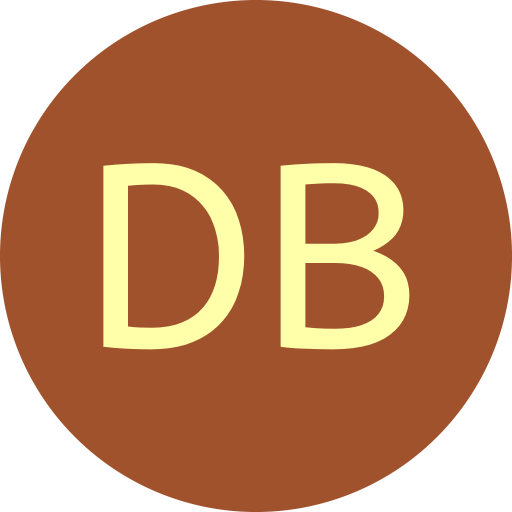#De Bruijn Sequence and Universal Cycle Constructions

$k$-ary Shift Rule Output
 Algorithm PCR1-Granddaddy PCR2-Grandmama PCR3 PCR4 PCR1 (alt) PCR2 (alt) PCR3 (alt) PCR4 (alt) Generic A1: f=a₁+1 mod k Generic A2: f=a₁+1 mod k Generic B1: f=a₁+1 mod k Generic B2: f=a₁+1 mod k Order $n$ Symbols $k$ 2 3 4 5 6 7 8 9
Constructing DB sequences using $k$-ary shift rules

Let $\Sigma_k = \{0,1,\ldots, k{-}1\}$. Let $\Sigma_k(n)$ denote the set of $k$-ary strings with length $n$. A function $f : \Sigma_k(n) \rightarrow \Sigma_k$ is a $k$-ary DB-successor if there exists a DB sequence $\mathcal{D}$ such that each string $\alpha = a_1a_2\cdots a_n \in \Sigma_k(n)$ is followed by the symbol $f(\alpha)$ in $\mathcal{D}$.

#### DB-successors based on the PCR

Using the PCR as the underlying feedback function, the four binary DB-successors are generalized to a larger alphabet [GSWW20], each in two different ways. These rules test in $O(n)$ time whether or not a string is a necklace, which is a string that is lexicographically smallest amongst all its rotations.

The following generalizes the binary Granddaddy DB-successor, the lexicographically least $k$-ary DB sequence. The rule is implicitly given in the proof of its corresponding concatenation construction [FM78], and also restated in [AAR+19b]. It can also be constructed via the Prefer-smallest greedy approach.

$k$-ary PCR1 Granddaddy DB-successor

If $\alpha = (k{-}1)^n$, let $j=n$; otherwise let $j$ be the smallest index of $a_2a_3\cdots a_n$ such that $a_j \ne k{-}1$. Let $x$ be the smallest symbol in $\Sigma_k$ such that $a_ja_{j+1}\cdots a_n x (k{-}1)^{j-2}$ is a necklace, or let $x=-1$ if no such symbol exists.

$f(\alpha) = \left\{ \begin{array}{ll} x &\ \ \mbox{if$x \geq 0$and$a_1 = k-1$;}\\ a_1{+}1 &\ \ \mbox{if$x \geq 0$and$a_1 \leq k-2$;}\\ {a_1} \ &\ \ \mbox{otherwise.}\end{array} \right.$

The following corresponds to the $k$-ary Grandmama DB-successor [DHS+18], which can also be constructed via a concatenation approach.

$k$-ary PCR2 Grandmama DB-successor

Let $j$ be the largest index of $a_2a_3\cdots a_n$ such that $a_j \ne 0$ or $j=1$ if no such index exists. Let $x$ be the largest symbol in $\Sigma_k$ such that $0^{n-j} x a_2\cdots a_{j}$ is a necklace, or let $x=-1$ if no such symbol exists.

$f(\alpha) = \left\{ \begin{array}{ll} 0 &\ \ \mbox{if$x \geq 0$and$a_1 = x$;}\\ a_1{+}1 &\ \ \mbox{if$x \geq 0$and$a_1 \leq x{-}1$;} \ \ \ \ \ \ \ \ \\ {a_1} \ &\ \ \mbox{otherwise.}\end{array} \right.$

The following generalizes the binary PCR3 DB-successor. It first corresponds to the shift rule in [SWW17].

$k$-ary PCR3 DB-successor

$f(\alpha) = \left\{ \begin{array}{ll} x{-}1 &\ \ \mbox{if$x \geq 0$and$a_1 = k{-}1$;}\\ a_1{+}1 &\ \ \mbox{if$x \geq 0$and$x{-}1 \leq a_1 \leq k{-}2$;}\\ {a_1} \ &\ \ \mbox{otherwise,}\end{array} \right.$

where $x$ is the smallest symbol in $\{1,2,\ldots, k{-}1\}$ such that $a_2a_3\cdots a_{n} x$ is a necklace, or $x=-1$ if no such symbol exists.

Perhaps the simplest $k$-ary DB successor to state (along with PCR3 above) generalizes the binary PCR4 DB-successor.

$k$-ary PCR4 DB-successor

$f(\alpha) = \left\{ \begin{array}{ll} 0 &\ \ \mbox{if$x \geq 0$and$a_1 = x{+}1$;}\\ a_1{+}1 &\ \ \mbox{if$x \geq 0$and$a_1 \leq x$;}\\ {a_1} \ &\ \ \mbox{otherwise,}\end{array} \right.$

where $x$ is the largest symbol in $\{0,1,\ldots, k{-}2\}$ such that $xa_2a_3\cdots a_{n}$ is a necklace, or $x=-1$ if no such symbol exists.

Each of the four binary DB-successors PCR1-PCR4 can be generalized in other ways [GSWW20].

Other works that present $k$-ary DB-successors include [ETZ86] and [YD93].

#### Generic constructions for arbitrary non-singular feedback functions

A more generic approach for any underlying non-singular feedback function, is available for download (the underlying feedback function is hard-coded). The option available for generation above uses the feedback function $f(a_1a_2\cdots a_n) = a_1 + 1 \bmod k$. This implementation is based on [GSWW20]. It generalizes both the Grandmama and the PCR3.

References

• [AAR+19b] G. Amram, Y. Ashlagi, A. Rubin, Y. Svoray, M. Schwartz, and G. Weiss. An efficient shift rule for the prefer-max De Bruijn sequence. Discrete Math., 342(1):226–232, 2019.

• [DHS+18] P. B. Dragon, O. I. Hernandez, J. Sawada, A. Williams, and D. Wong. Constructing de Bruijn sequences with co-lexicographic order: the $k$-ary Grandmama sequence. European J. Combin., 72:1-11, 2018.

• [Etz86] T. Etzion. An algorithm for generating shift-register cycles. Theoret. Comput. Sci., 44(2):209-224, 1986.

• [GSWW20] D. Gabric, J. Sawada, A. Williams, and D. Wong. A successor rule framework for constructing $k$-ary de Bruijn sequences and universal cycles. IEEE Transactions on Information Theory, 66(1):679–687, 2020.

• [FM78] H. Fredricksen and J. Maiorana. Necklaces of beads in $k$ colors and $k$-ary de Bruijn sequences. Discrete Math., 23(3):207-210, 1978.

• [SWW17] J. Sawada, A. Williams, and D. Wong. A simple shift rule for $k$-ary de Bruijn sequences. Discrete Math., 340(3):524-531, 2017.

• [YD93] J.-H. Yang and Z.-D. Dai. Construction of $m$-ary de Bruijn sequences (extended abstract). In J. Seberry and Y. Zheng, editors, Advances in Cryptology | AUSCRYPT '92, pages 357-363, Berlin, Heidelberg, 1993. Springer Berlin Heidelberg.